Next: Worked example 12.4: Halley's Up: Orbital motion Previous: Worked example 12.2: Acceleration

## Worked example 12.3: Circular Earth orbit

Question: A satellite moves in a circular orbit around the Earth with speed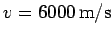. Determine the satellite's altitude above the Earth's surface. Determine the period of the satellite's orbit. The Earth's mass and radius are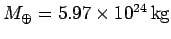and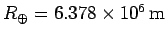, respectively.

Answer: The acceleration of the satellite towards the centre of the Earth is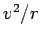, whereis its orbital radius. This acceleration must be provided by the acceleration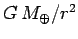due to the Earth's gravitational attraction. Hence,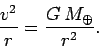The above expression can be rearranged to giveThus, the satellite's altitude above the Earth's surface is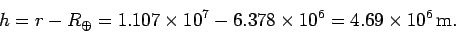The satellite's orbital period is simply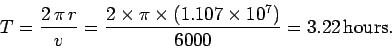Richard Fitzpatrick 2006-02-02